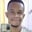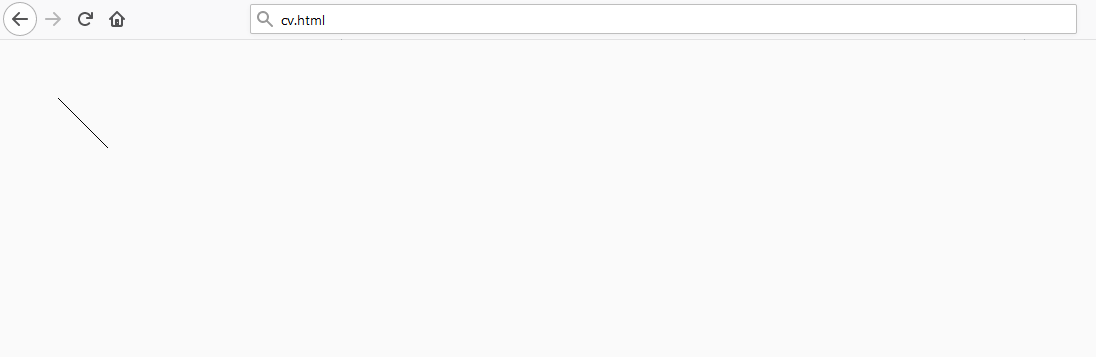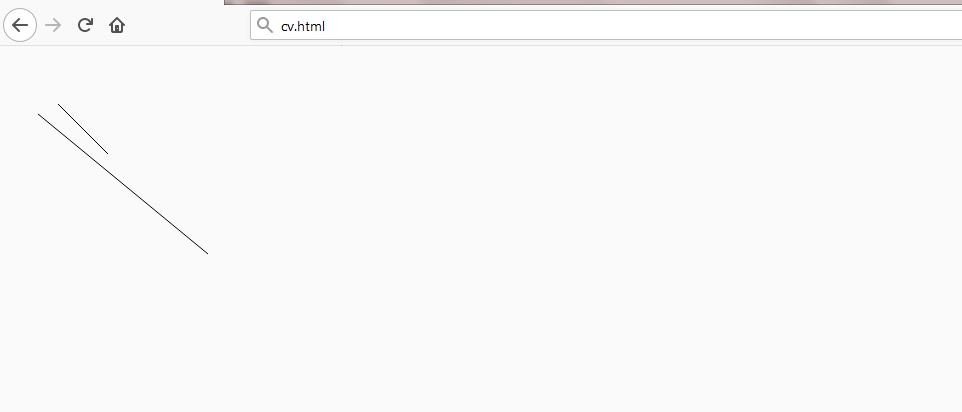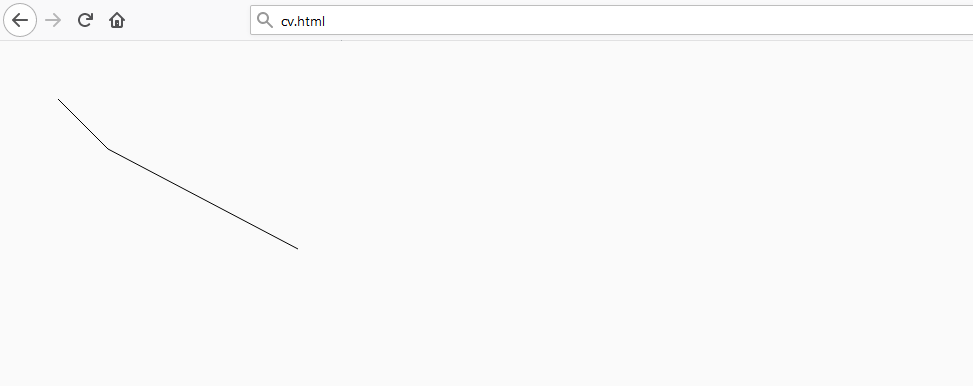Trusted answers to developer questions
Trusted Answers to Developer Questions

Related Tags

canvas
javascript
communitycreator

# How to draw lines and paths in HTML CanvasChidume Nnamdi

Grokking Modern System Design Interview for Engineers & Managers

Ace your System Design Interview and take your career to the next level. Learn to handle the design of applications like Netflix, Quora, Facebook, Uber, and many more in a 45-min interview. Learn the RESHADED framework for architecting web-scale applications by determining requirements, constraints, and assumptions before diving into a step-by-step design process.

### Overview

Lines are drawn by joining two points on the canvas. A path is a list of points, connected by segments of lines that can be of different shapes (curved or not), widths, and colors. A path, or even a subpath, can be closed.

### Syntax

To make shapes with paths, we need the following APIs:

• beginPath(): Begins a path on the canvas.

• closePath(): Ends the path created by beginPath.

• moveTo(x, y): Moves the cursor to the $x$ and $y$ position on the canvas grid.

• lineTo(x, y): Marks a path or line beginning from the $x,y$ position specified by the moveTo method to the $x,y$ position specified by lineTo.

• stroke(): Outlines the marked path(s) withlineTo and moveTo.

• fill: Fills the content area of the path(s) marked by lineTo.

To draw a single line, you need the following commands:

context.beginPath()
context.moveTo(50, 50)
context.lineTo(100, 100)
context.stroke()


### Explanation

• First, we create the path with beginPath.

• We set the drawing pen/cursor at the $x,y$ = $(50,50)$ postion.

• Then, we use the lineTo method to draw a line. We tell it to mark a line beginning from $x,y$ = $(50,50)$, set by moveTo, to $x,y$ = $(100,100)$. context.stroke() draws the outline set by the previous lineTo(100,100). context.stroke() draws the line from $x,y$ = $(50,50)$ to $x,y$ = $(100,100)$.As shown above, the line is visible in the browser. Without the stroke() call, we won’t see the line. The color of the line is black, which is the default of the canvas when no color is set.

### Example

We can draw lines on the canvas by beginning and closing paths.

context.beginPath()
context.moveTo(50, 50)
context.lineTo(100, 100)
context.stroke()
context.closePath()

context.beginPath()
context.moveTo(30, 60)
context.lineTo(200, 200)
context.stroke()
context.closePath()


### Explanation

The code above draws two lines in our browser, the first line from our previous example and a new line.

• Before you begin the new line, you have to close the path of the last line and begin a new one.
• Use moveTo to move the pen to $x,y$ = $(30, 60)$ as the starting point.
• Then, you can mark a line from there to (x,y) = $(200,200)$.
• We use stroke() to draw out the outline.As shown above, the two lines are visible in the browser.

### Example

We can draw and connect lines to form complex shapes.

context.beginPath()
context.moveTo(50, 50)
context.lineTo(100, 100)
context.lineTo(290, 200)
context.stroke()
context.closePath()


The code above will draw the following shape:### Explanation

• moveTo(50,50) sets the pen at position $x,y$ = $(50,50)$.
• Then, lineTo(100,100) marks a line from $x,y$ = $(50,50)$ to $(x,y)$ = $(100,100)$.
• lineTo(290,200) traces the line from $x,y$ = $(100,100)$, until eventually the line reaches $x,y$ = $(290, 200)$. This forms the shape we see in our browser.

Note: Please refer to the following shots for information related to this topic:
Drawing triangles
Filling paths
Drawing rectangles
Drawing circles and arcs

RELATED TAGS

canvas
javascript
communitycreator

CONTRIBUTORChidume Nnamdi

Grokking Modern System Design Interview for Engineers & Managers

Ace your System Design Interview and take your career to the next level. Learn to handle the design of applications like Netflix, Quora, Facebook, Uber, and many more in a 45-min interview. Learn the RESHADED framework for architecting web-scale applications by determining requirements, constraints, and assumptions before diving into a step-by-step design process.

Keep Exploring

Learn in-demand tech skills in half the time